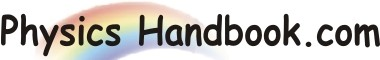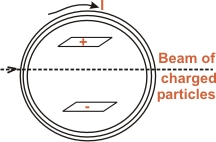HOME TOPICS DEFINITIONS TABLES LAWS INVENTIONS EXPERIMENTS QUIZ VIDEOS
 A B C D E F G H I J K L M N O P Q R S T U V W X Y Z
Cross Fields
If a uniform electric field and a uniform magnetic field are perpendicular to each other in such a way that they produce deflection of charged particles (e.g. a beam of electrons in the opposite senses, they are called Cross Fields.
Thus referring to Fig, the uniform electric field set up by charged parallel-plate capacitor and uniform magnetic field produced by a current carrying coil are perpendicular to each other. Further, these fields would produce deflections of a beam of charges particles in the opposite direction. Hence the system constitutes cross fields. If a beam of charged particles (each carrying a charge q) moving with a velocity v is subjected to cross fields, then,

Magnetic force on charged particle, Fm = q v B
Electric force on charged particle, Fe = q E

The two forces act in opposite direction. If the fields are so adjusted that the two forces are of the same magnitude, then,
q v B = q E or v = E / BUnder such conditions, the beam will go unperfected. The cross fields find many applications in physics. Once important application of cross fields in the determination of e/m (change-to-mass ratio) of an electron.